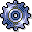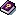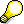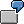Plan Price CalculationUse

Plan price calculation determines prices for the plan activity types of each cost center and activity type or business process (see also:Actual Price Calculation).

In the plan, the SAP System considers all the planned activity relationships between cost centers and between business processes. It calculates the prices iteratively by dividing the plan costs by the plan activity quantity.

Alternatively, you can also determine the fixed portion of the price as the quotient (ratio) of plan costs and capacity. This is useful when the costing of a given product is not to be influenced by the costs of providing the maximum activity quantity. For example, an energy-supplying cost center must always be capable of supplying the maximum level of activity, even if this activity quantity is not always used. In this case, the fixed provision costs should remain on the supplying cost center, since the full amount cannot be assigned directly to the product costs.You can only execute price calculation if, during the activity type planning for the given cost centers or business processes, you have set theprice indicator to 1 or 2 . Price calculation is based on the plan activity quantity if you select "1", or based on the capacity if you select "2". It is not advisable to carry out price calculation if you have specified all prices manually (price indicator "3").

Prerequisites

For iterative price calculation all necessary data (cost of all business transactions, primary and secondary costs, activity-dependent and activity-independent costs) must be available. This requires that the following conditions have been met:

1. Planning is complete.

2. If orders or logistics are part of planning you must settle the orders or projects to the cost centers.

Features

The SAP System calculates different prices, depending on the Methods indicator, which you set in the corresponding version. To set this indicator, call up the Implementation Guide (IMG) for Cost Center Accounting or Activity-Based Costing, then choose Planning ® Basic Settings ® Maintain Versions. Select the version you require and choose Settings for fiscal year. Select the corresponding fiscal year and choose Detail. You can set the Method you require under Plan.

The following values are possible:

 1 Periodic prices 2 Average prices 3 Cumulative prices

For more information, seeMethods for Price Calculation.

The SAP System calculates the price of an activity type on a cost center or on a business process by dividing the sum of all the costs of this cost center or business process by the plan activity or capacity. As a result, different periodic prices can occur when you use the Periodic price method, which means that either the total plan costs of a cost center or business process, or the plan activity, or both of these factors, can display different values. The following factors produce these periodic differences.

• Distribution keys

If you do not distribute the plan costs and activity equally between the periods, this results in differing values for plan activity and plan costs (primary and secondary), and therefore differing tracing factors in price calculation.

• Equivalence numbers

You can plan equivalence numbers by period. Different equivalence numbers in individual periods result in higher portions of activity-independent costs being distributed to the activity types in these periods. This results in differing total costs in the individual periods, which means that the fixed portion of the price changes periodically. Equivalence numbers have the value 1 unless another value is entered. This means that all activity types are weighted equally.

In Cost Center Accounting, you include activity-independent plan costs in your activity prices by using splitting rules which you specify as part of plan cost splitting (for more information, see: plan cost splitting). The SAP System distributes the activity-independent plan costs of a cost center to the activity types of the cost center. The simplest way to do this is to distribute on the basis of equivalence numbers. The SAP System calculates the ratio of the equivalence number of one activity type to the total of all equivalence numbers for activity types on the cost center. The activity-independent plan costs are multiplied by this weighting factor and added to the activity-dependent plan costs.

Activity-independent plan costs are always fixed. Therefore, the plan fixed quotient of the activity-dependent plan costs is increased by the amount which results from the distribution of the activity-independent plan costs based on equivalence numbers.You have planned \$30,000 activity-independent costs on cost center A. You have also planned the activity types as follows.

 Activity type Quantity Equivalence number FST 400 hrs 2 REP 100 hrs 1 Total 3

The activity-independent plan costs are then incorporated in the price calculation:

 Activity type Fixed costs by activity type Fixed activity portion FST \$30,000 X 2/3 = \$20,000 \$20,000 /400 hrs = \$50 /hr REP \$30,000 X 1/3 = \$10,000 \$10,000 /100 hrs = \$10 /hr

The activity-independent portion of the price for cost center A is \$50/hr for activity type FST and \$10/hr for activity type REP.Plan cost splitting is automatically performed in plan price calculation. Separate plan cost splitting is necessary only if you want to review the splitting process using the explanation feature.Price Calculation

You have planned your data as follows.

 Cost Center 1 Cost Center 2 Activity type REP FST Plan activity 1,000 hrs 100 hrs Primary costs Wages \$10,000 \$5,000 Salaries \$5,000 \$5,000 Energy \$1,000 \$1,000 Total primary costs \$16,000 \$11,000 Secondary costs Activity from: Cost Center 2 Cost Center 1 10 hrs 50 hrs

The SAP System calculates the prices for these cost centers step by step.

1. The primary costs are divided by the plan activity. The resulting price is used to valuate the activity input.
2. In the following steps, the calculated prices are used to valuate other activity relationships. The exact price of an activity type of a cost center is determined though iteration.

Instead of using the plan activity, you can also use the capacity (maximum activity quantity) as the basis for calculating the fixed portion of the iterative prices. You make these settings during activity type planning (see: Activity Type Planning). Note that the capacity of a cost center for a given activity type can only be used for fixed costs as the basis for price calculation. The plan activity is used as the basis for the variable costs, even if the price indicator is set to "2" (price calculation based on capacity).A cost center supplying energy must always be ready to supply the maximum amount of activity. The costs for this activity remain constant since they are based on the capacity.Once all prices have been calculated, the SAP System revaluates the activity relationships between the cost centers.